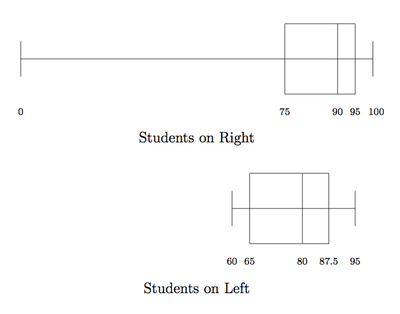# Comparing Test Scores

Alignments to Content Standards: 6.SP.B.4 6.SP.B.5.c

In Mrs. Sanchez' math classroom, more people sit on the right-hand side of the room than the left.  The students on the right-hand side of the classroom received the following scores on an exam worth 100 points:

$$85,\, 90,\, 100,\, 95,\, 0,\, 0,\, 90,\, 70,\, 100,\, 95,\, 80,\, 95$$

The students on the left received these test scores:

$$65,\, 80,\, 90,\, 65,\, 80,\, 60,\, 95,\, 85$$

1. Make two box plots of the students' scores, one for each side of the room.
2. Make a statistical argument that the students on the right-hand side were more successful.
3. Make a statistical argument that the students on the left-hand side were more successful.

## IM Commentary

The goal of this task is to critically compare the center and spread of two data sets. Although the mean is not specifically requested, it can be used as an argument in favor of the left's performance. The teacher may wish to request or suggest for students to calculate the mean or tell them that the statistical arguments for (b) and (c) should not just be based on the box plots.  The teacher will also want to make sure that the two box plots can be directly compared so the values on the two number lines of the boxplots need to correspond to one another.

Statistics is a powerful tool for supporting arguments in a variety of contexts: medicine, education, sports, and ecology to name a few. There are usually choices to make, however, both in which statistics to use and how they are reported. This task gives students an opportunity to think carefully about how to analyze the data in order to support a particular point of view.

This task is based on an idea used for a worksheet developed for one of the UCLA Curtis Center's Saturday trainings given on December 6, 2014. In that problem, two students' quiz scores were compared and the structure of the numbers were very similar.

## Solution

1. The box plots for the two halves of the room are shown together to make them easier to compare:The left whisker is the lowest score, 0 for the right-hand side and 60 for the left-hand side. The right whisker is the highest score, 100 for the right-hand side and 95 for the left-hand side. For the other values, the scores are listed in increasing order, (0,0,70,80,85,90,90,95,95,95,100,100) for the right-hand side. There are twelve scores and the middle two are both 90's so the median is 90. The middle two values for the first half of the data, (0,0,70,80,85,90), are 70 and 80 so the first quartile is 75. The middle two values for the second half of the data are both 95's so the third quartile is 95, and similar for the other plot.

2. There are many ways to argue that the "righties" (the students on the right-hand side) performed better on this test than the "lefties," including:

• Two righties got perfect scores of 100 while none of the lefites did.
• The box plot shows that more than half of the righties got 90 percent. Only a quarter of the lefties did this well on the test.
• If 70 percent is a passing score, only two righties did not pass (probably because they missed the test) while three of eight lefties did not pass the test.
3. Some arguments for why the lefties performed better than the righties on this test include:

• The lowest score of the lefties on the test was 60, which is much higher than the lowest of the righties' scores, 0.
• The average (mean) of the lefties' scores is 77.5 which is higher than the average of the righties' scores, 75.
• Consistency is good and there is much less variation in the lefties' scores than in the righties.